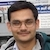# NAEST 2015 Screening Test: Bottle with a HoleBy

A water bottle has a hole near its bottom. The water flows out of the hole when the bottle's cap is open but it stops flowing out when the cap is closed. Why?

Question: Why the water is not coming out of the hole when the cap is closed but it starts coming out when the cap is removed?

Solution: We need to rope in concepts related to atmospheric pressure, hydrostatic pressure and pressure change due to gaseous expansion to get the answer. Let $\rho$ be the density of water and $h$ be the height of water column (above the hole).Let us consider the case when the cap is open. In this case, the air inside the bottle (above the water surface) is connected to the atmospheric air. Thus, the pressure just above the water surface is equal to the atmospheric pressure $p_0$. The pressure inside the water at the level of hole is given by \begin{align} p_\text{in}=p_0+\rho g h \end{align} where $\rho g h$ is the hydrostatic pressure due to the water column of height $h$.

The pressure outside the hole is $p_0$ i.e., \begin{align} p_\text{out}=p_0 \end{align} The pressure difference $p_\text{in}-p_\text{out}=\rho g h$ makes the water flow out of the bottle.

Now, consider the case when the cap is closed. In this case, the air inside the bottle is not connected to the atmospheric air. Careful observation reveals that a few drops of water come out of the hole in the beginning. This decreases the volume of water and increases the volume of trapped air. The increase in air volume (air expansion) decreases air pressure. Thus, pressure above the water surface is not equal to $p_0$ but it is less than that. Actually, the air pressure in this case is \begin{align} p_\text{air}=p_0-\rho g h. \end{align} The pressure inside and outside the hole is equal to the atmospheric pressure. Thus, water does not flow in this case.

If the hole size is very small then water does not come out even if the cap is opened. You can check it yourself. This happens due to surface tension. The surface tension becomes significant when the hole size is small. It prevents the water to come out of the hole.